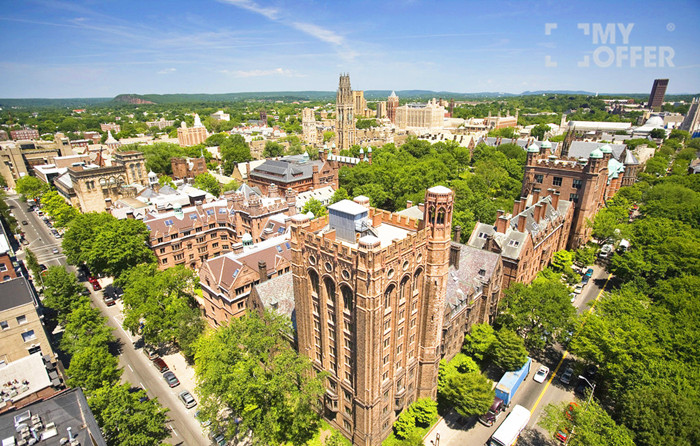2018世界大学排名 2017世界大学排名 学校名称 =3 2 加州理工学院 =3 3 斯坦福大学 5 5 麻省理工学院 6 6 哈佛大学 7 7 普林斯顿大学 9 =10 芝加哥大学 =10 13 宾夕法尼亚大学 12 12 耶鲁大学 13 17 约翰霍普金斯大学 14 16 哥伦比亚大学 15 14 加州大学洛杉矶分校 17 18 杜克大学 18 =10 加州大学伯克利分校 19 19 康奈尔大学 20 20 西北大学 21 21 密歇根大学 24 23 卡耐基梅隆大学 =25 =25 华盛顿大学 =27 32 纽约大学 31 41 加州大学圣地亚哥分校 33 =33 佐治亚理工学院 37 =36 伊利诺伊大学香槟分校 43 45 威斯康辛大学麦迪逊分校 49 50 德克萨斯大学奥斯汀分校 =50 =51 布朗大学 =50 =57 圣路易斯华盛顿大学 53 48 加州大学圣塔芭芭拉分校 =54 =51 加州大学戴维斯分校 =56 53 明尼苏达大学 =56 56 北卡罗来纳大学教堂山分校 60 70 普渡大学 66 =60 南加州大学 69 67 马里兰大学帕克分校 =70 64 波士顿大学 =70 =72 俄亥俄州立大学 77 68 宾夕法尼亚州立大学 =83 101 密歇根州立大学 =86 87 莱斯大学 89 =82 达特茅斯学院 98 =82 埃默里大学 99 =98 加州大学欧文分校 =100 =116 科罗拉多大学波尔得分校 =100 =80 匹兹堡大学